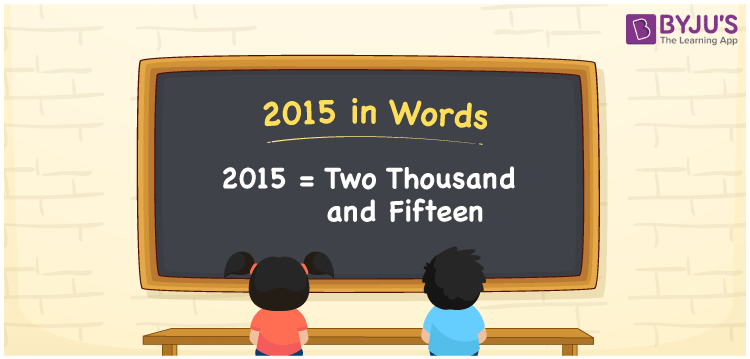# 2015 in Words

We can write 2015 in words as Two thousand and fifteen. We can easily derive the word form of 2015 using a place value chart. Word form of 2015 is nothing but the number name for 2015. For example, if you find the number 2015 on a calendar, you may read it as “Two thousand and fifteen” or “Two thousand fifteen”. Similarly, we can write all cardinal numbers in words.

 2015 in words Two thousand and fifteen Two thousand and fifteen in Numbers 2015

## 2015 in English Words

We generally write numbers in words using the English alphabet. Thus, we can read 2015 in English as “Two thousand and fifteen”.## How to Write 2015 in Words?

Let’s understand how to derive the number 2015 in words using a place value chart. The below table represents the place values for all the four digits of the number 2015.

 Thousands Hundreds Tens Ones 2 0 1 5

Here, ones = 5, tens = 1, hundreds = 0, and thousands = 2.

Thus, we can write the expanded form as:

2 × Thousand + 0 × Hundred + 1 × Ten + 5 × One

= 2 × 1000 + 0 × 100 + 1 × 10 + 5 × 1

= 2000 + 10 + 5

= Two thousand + Fifteen {since ten + five = fifteen}

= Two thousand and fifteen

Hence, 2015 in words is written as Two thousand and fifteen.

2015 is a natural number that precedes 2016 and succeeds 2014.

2015 in words – Two thousand and fifteen

Is 2015 an even number? – No

Is 2015 an odd number? – Yes

Is 2015 a prime number? – No

Is 2015 a composite number? – Yes

Is 2015 a perfect square number? – No

Is 2015 a perfect cube number? – No

## Frequently Asked Questions on 2015 in Words

Q1

### How do you write the year 2015 in words?

We can write the year 2015 in words as Two thousand and fifteen.
Q2

### Write 16 September 2015 in words.

16 September 2015 in words is written as “Sixteen September Two thousand and fifteen”.
Q3

### Express the amount Rs. 2015 in words.

The amount Rs. 2015 can be expressed in words as “Two thousand and fifteen rupees”.